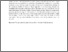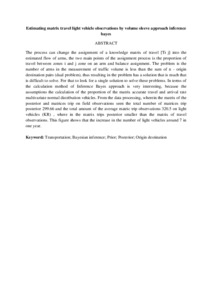# Estimating matrix travel light vehicle observations by volume sleeve approach inference bayes

## Citation

Abusini, Sobri and Kilicman, Adem (2016) Estimating matrix travel light vehicle observations by volume sleeve approach inference bayes. Applied Mathematics & Information Sciences, 10 (5). pp. 1735-1738. ISSN 1935-0090; ESSN: 2325-0399

## Abstract

The process can change the assignment of a knowledge matrix of travel [Ti j] into the estimated flow of arms, the two main points of the assignment process is the proportion of travel between zones i and j zone on an arm and balance assignment. The problem is the number of arms in the measurement of traffic volume is less than the sum of n - origin destination pairs (dual problem), thus resulting in the problem has a solution that is much that is difficult to solve. For that to look for a single solution to solve these problems. In terms of the calculation method of Inference Bayes approach is very interesting, because the assumptions the calculation of the proportion of the matrix accurate travel and arrival rate multivariate normal distribution vehicles. From the data processing, wherein the matrix of the posterior and matrices trip on field observations seen the total number of matrices trip posterior 299.66 and the total amount of the average matric trip observations 320.5 on light vehicles (KR) , where in the matrix trips posterior smaller than the matrix of travel observations. This figure shows that the increase in the number of light vehicles around 7 in one year.Preview
Text
Estimating matrix travel light vehicle observations by volume sleeve approach inference bayes.pdfView Item